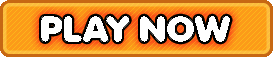Bookmark

# Freddy Football Basic Math Facts Addition, Subtraction, Multiplication, Division

4.3 stars (1,940 plays / 19 votes)Freddy Football | Basic Math Facts (Addition, Subtraction, Multiplication, & Division)Answer math facts to help Freddy dodge tacklers and kick field goals.
4.3 stars out of 5 (1,940 plays / 19 votes)

KidzSearch > Games > Math GamesTags:  math    basic    fact    addition    subtraction    multiplication    multiply    division    divide    timed    math    problem    elementary    math    number    sense    football    sport    integer    negative    positive    fourth    fifth    sixthMember of the American Library Association  Copyright 2005-2020 KidzSearch.com